Start typing, then use the up and down arrows to select an option from the list.# Calculate Oxidation Numbers Example 3

Jules Bruno
169views
2
here. In this example, it says which compound has oxygen with lowest oxidation state? If we take a look at the first one, we have n a 02 We have one group on a element with two oxygen's. Remember, that pattern tells us we have a super oxide, and if you have a super oxide, that means the oxidation number of oxygen is minus a half for the next one. We have CO two now. It's not a super oxide. For to be a super oxide, we would need one group one a element with the two. Oxygen's carbon is not in Group One A. It's a group for a so it's not a super oxide. Next, it's not a peroxide, either. To be a peroxide, you need to group one elements with two oxygen's, so it's not a peroxide. It's not a super oxide. Therefore, oxidation number is minus two. For the next one, we have CS 202 That's two Sessoms, which are Group one A with two oxygen's that fits the definition of a peroxide. So this is a session peroxide, so with oxidation, number for oxygen would be minus one. And then finally we have 02 This is the standard or natural state of oxygen. Remember, an element found in its standard or natural state has an oxidation number of zero. So out of all our choice is the one that has the lowest oxidation number. The one with the most negative value would be be CO two. So just remember, if we have specific rules for that given element, utilize them to find the correct answer.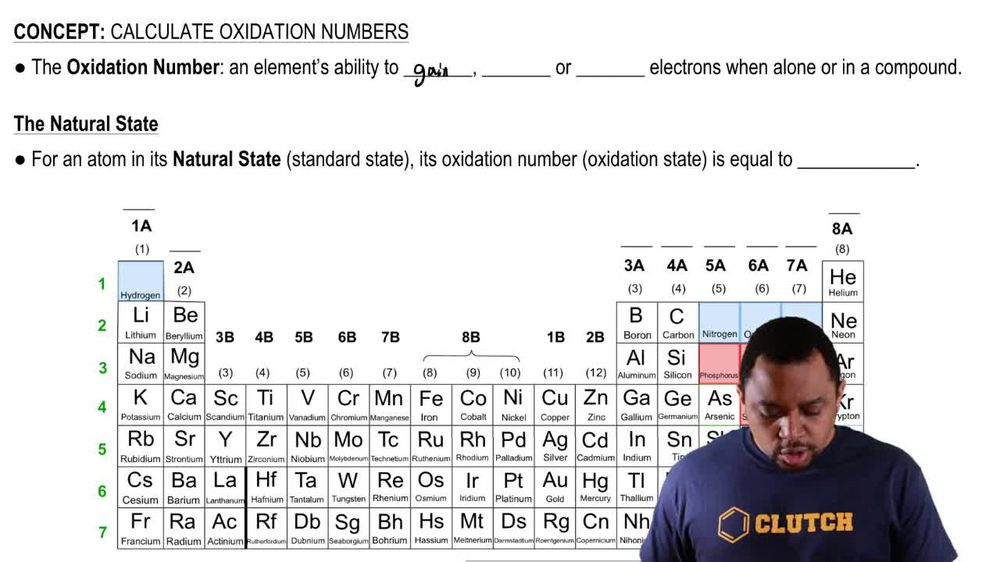02:42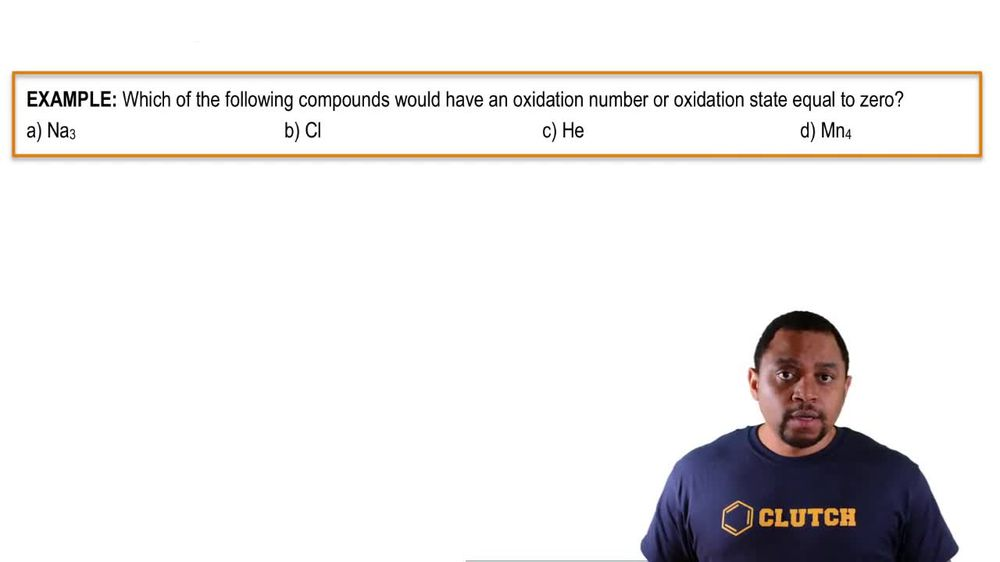01:16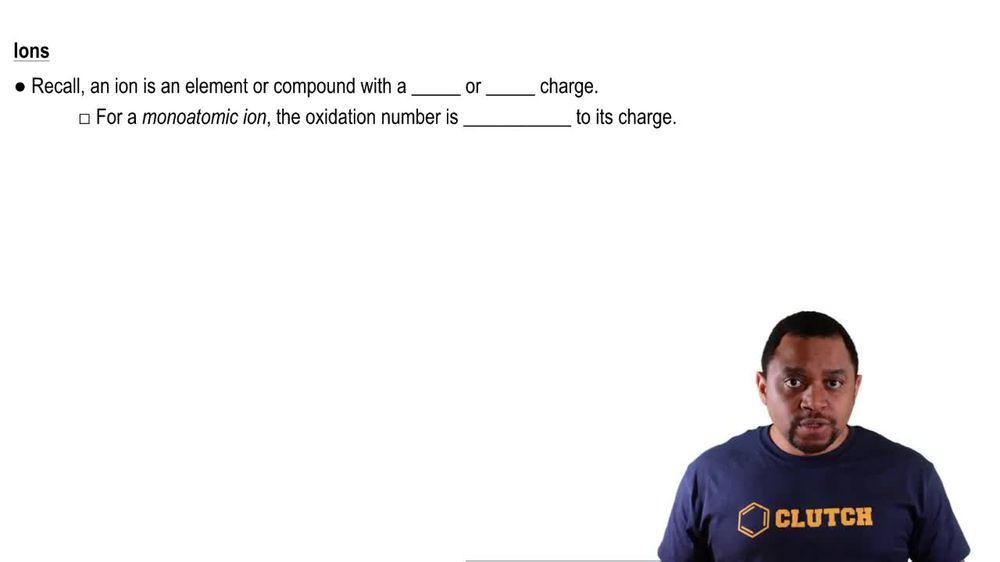01:15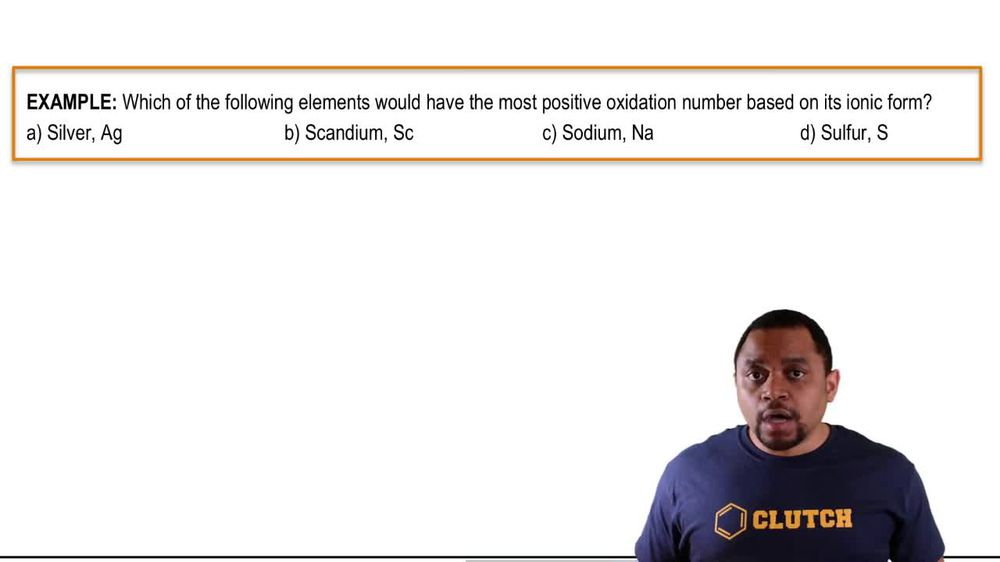01:38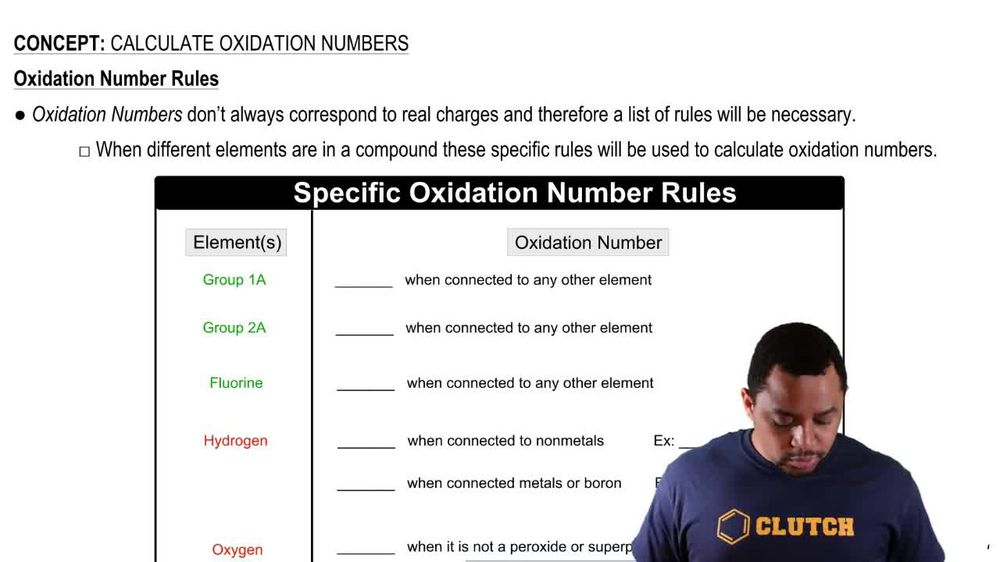03:13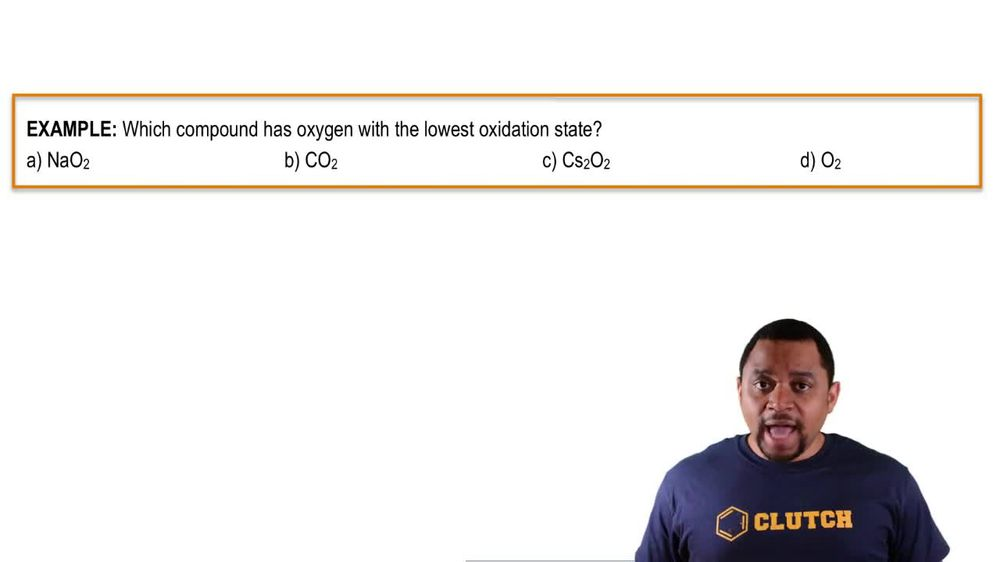01:36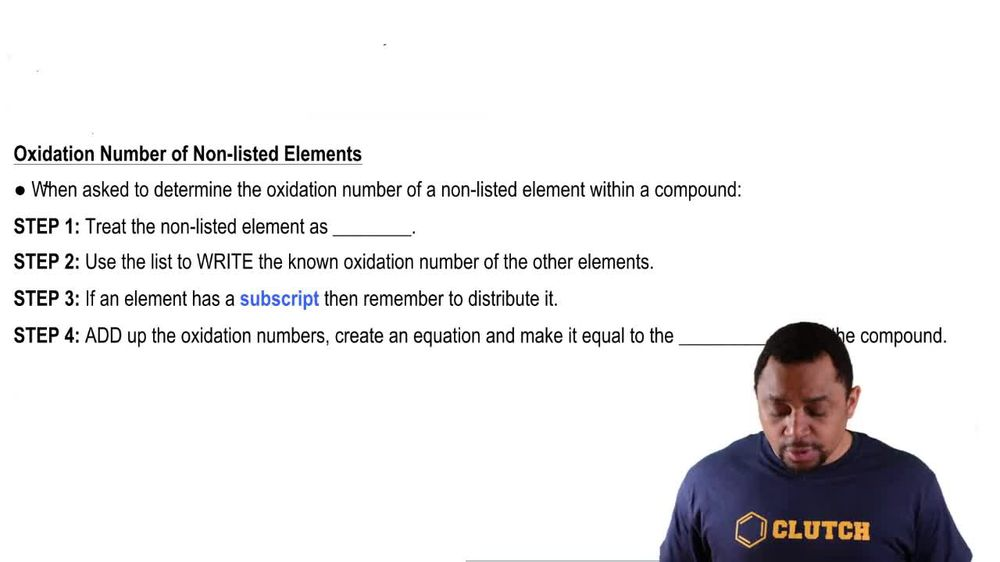01:09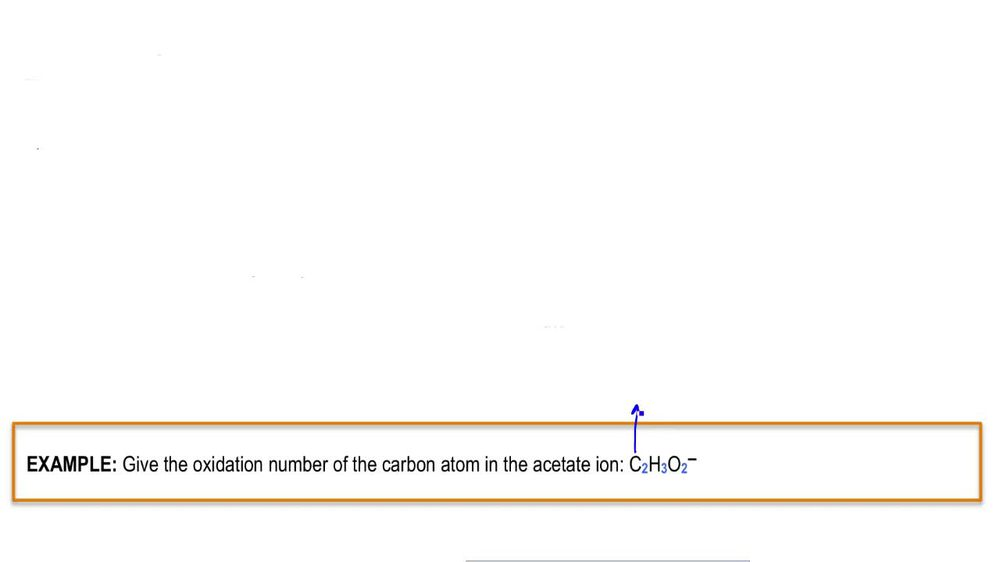01:22